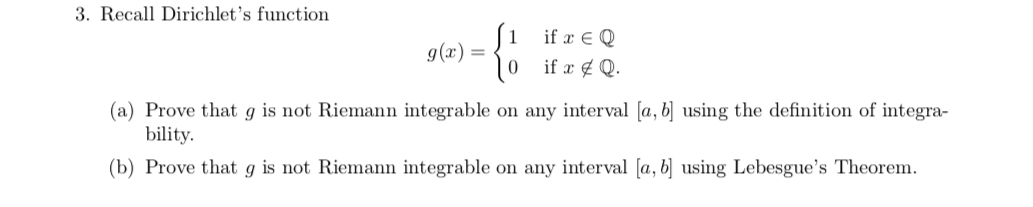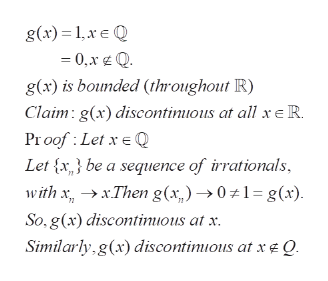# 3. Recall Dirichlet's functionif E Qg(x)(a) Prove that g is not Riemann integrable on any interval [a, b] using the definition of integra-bility(b) Prove that g is not Riemann integrable on any interval [a, bj using Lebesgue's Theorem.

Questionhelp_outlineImage Transcriptionclose3. Recall Dirichlet's function if E Q g(x) (a) Prove that g is not Riemann integrable on any interval [a, b] using the definition of integra- bility (b) Prove that g is not Riemann integrable on any interval [a, bj using Lebesgue's Theorem. fullscreen
check_circleExpert Solution
Step 1

(b) To prove that the given function is not Riemann integrable using Lebesgue;s theorem

Step 2help_outlineImage Transcriptioncloseg(x) 1xQ 0,x Q g(x) is bounded (throughout R) Claim g(x) discontinious at all x R. Proof Let x Q Let x} be a sequence of irrationals with xxThen g(x,)01= g(x) So,g(x) discontinious at x Simiarly g(x)discontimious at xeQ. fullscreen

### Want to see the full answer?

See Solution

#### Want to see this answer and more?

Solutions are written by subject experts who are available 24/7. Questions are typically answered within 1 hour*

See Solution
*Response times may vary by subject and question
Tagged in

### Math